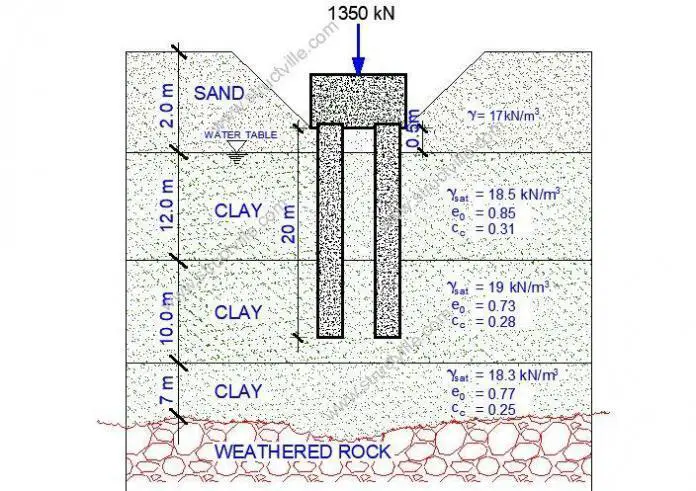When a group of piles are founded on saturated clays, the pile group undergoes time-dependent consolidation settlement. This can be calculated using the consolidation settlement equations, and generally involves evaluating the increase in stress when the soil beneath a pile group is subjected to pressure due to load from the superstructure/column Qg. The consolidation settlement of pile groups can be estimated by assuming an approximate distribution method that is commonly referred to as the 2:1 method.

The calculation procedure involves the following steps as described in Das (2008);

1. Obtain the total length of embedment of the pile L, and the total load (service load) transmitted from the column to the pile cap (Qg).
2. The load Qg is assumed to act on a fictitious footing depth of 2L/3 from the top of the pile. The load Qg spreads out along 2 vertical:1 horizontal lines from this depth.
3. Calculate the effective stress increase caused at the middle of each soil layer by the load Qg:

∆σi‘ = Qg/[(Bg + zi) (Lg + zi)]

Where;
∆σi‘ = effective stress increase at the middle of layer i
Bg, Lg = Width and length of the plan of pile group, respectively
zi = distance from z = 0 to the middle of the clay layer, i

4. Calculate the settlement of each layer caused by the increased stress:

∆Sc(i) = [∆e/ (1 + e0(i)] × Hi

where;
∆Sc(i) = consolidation settlement of layer i
∆ei = change of void ratio caused by the stress increase in layer i
e0(i) = initial void ratio of layer i (before construction)
Hi = Thickness of layer i

5. Calculate the total consolidation settlement of the pile group by;
∆Sc(g) = ∑∆Sc(i)

Solved Example
Calculate the consolidation settlement of a group of 4 piles founded on layers of clay as shown below. The piles are 600mm in diameter and forms a square plan of 1800mm x 1800mm. The service load from the column on the pile is 1350 kN.

Solution
Depth of fictitious footing = 2L/3 = 2(20)/3 = 13.33 m
By implication, it can be seen that the influence depth went beyond the first layer of clay. Therefore, consolidation settlement calculation commences from the second clay layer of the soil profile. Let us therefore call this first layer of influence, layer 1.

Layer 1
∆σ1‘ = Qg/[(Bg + z1) (Lg + z1)] = 1350 / [(1.8 + 4.585) × (1.8 + 4.585)] = 33.114 kPa

σ0(1)‘ = (2 × 17) + 12(18.5 – 9.81) + 5.415(19 – 9.81) = 188.04 kPa

∆Sc(1) = [(Cc(1)H1)/(1 + e0)] × log[(σ0(i)‘ + ∆σi‘ )/σ0(i)‘]
∆Sc(1) = [(0.28 × 9.17)/(1 + 0.73)] × log[(188.04 + 33.114)/188.04] = 0.1045m = 104.473 mm

Layer 2
∆σ2‘ = Qg/[(Bg + z2) (Lg + z2)] = 1350 / [(1.8 + 12.67) × (1.8 + 12.67)] = 6.4475 kPa

σ0(2)‘ = (2 × 17) + 12(18.5 – 9.81) + 10(19 – 9.81) + 3.5(18.3 – 9.81) = 259.895 kPa

∆Sc(2) = [(Cc(2)H2)/(1 + e0)] × log[(σ0(2)‘ + ∆σ2‘ )/σ0(2)‘]
∆Sc(2) = [(0.25 × 7)/(1 + 0.77)] × log[(259.895 + 6.4475)/259.895] = 0.0104m = 10.48 mm

Therefore total settlement ∆Sc(g) = ∆Sc(1) + ∆Sc(2) = 104.473 + 10.48 = 114.953 mm

Thank you so much for visiting Structville today, and God bless you.

References
Das B.M. (2008): Fundamentals of Geotechnical Engineering (3rd Edition). Cengage Learning, USA

#### 1 COMMENT

1. Thank you very much for this knowledge.
We learn everyday.